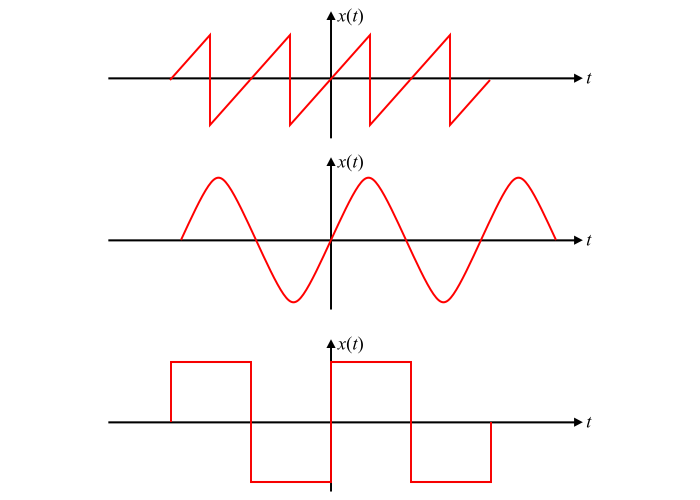# Signals and Systems â€“ What is Odd Symmetry?

## Importance of Wave symmetry

If a periodic signal $x(t)$ has some type of symmetry, then some of the trigonometric Fourier series coefficients may become zero and hence the calculation of the coefficients becomes simple.

## Odd or Rotation Symmetry

When a periodic function $x(t)$ is antisymmetric about the vertical axis, then the function is said to have the odd symmetry or rotation symmetry.

Mathematically, a function $x(t)$ is said to have odd symmetry, if

$$\mathrm{x(t)=-x(-t)… (1)}$$

Some functions having odd symmetry are shown in the figure. It is clear that the odd symmetric functions are always antisymmetrical about the vertical axis.## Explanation

As we know that any periodic signal $x(t)$ can be split into even and odd components, i.e.,

$$\mathrm{x(t)=x_{e}(t)+x_{0}(t)… (2)}$$

If the function $x(t)$ is an odd function, then,

$$\mathrm{x_{e}(t)=0}$$

$$\mathrm{\therefore\:x(t)=x_{0}(t)… (3)}$$

The trigonometric Fourier coefficients of the function can be evaluated as follows −

The coefficient $a_{0}$ is given by,

$$\mathrm{a_{0}=\frac{1}{T} \int_{−T/2}^{T/2}x(t)\:dt=\frac{1}{T}\int_{−T/2}^{T/2}x_{0}(t)\:dt}$$

For an odd function, the area under the curve over one period is zero, i.e.,

$$\mathrm{\int_{−T/2}^{T/2}x_{0}(t)\:dt=0}$$

$$\mathrm{\therefore\:a_{0}=0… (4)}$$

The coefficient $a_{n}$ is given by,

$$\mathrm{a_{n}=\frac{2}{T} \int_{−T/2}^{T/2}x(t)cos\:n\omega_{0} t\:dt}$$

$$\mathrm{\Rightarrow\:a_{n}=\frac{2}{T} \int_{−T/2}^{T/2}x_{0}(t)cos\:n\omega_{0} t\:dt}$$

Since the function $(x_{0}(t)\:cos\:n\omega_{0đť‘ˇ}t)$ is an odd function, hence its integral over one complete is zero.

$$\mathrm{\therefore\:a_{n}=0… (5)}$$

And the coefficient $b_{n}$ is given by,

$$\mathrm{b_{n}=\frac{2}{T}\int_{−T/2}^{T/2}x(t)sin\:n\omega_{0}t\:dt}$$

$$\mathrm{\Rightarrow\:b_{n}=\frac{2}{T}\int_{−T/2}^{T/2}x_{0}(t)sin\:n\omega_{0}t\:dt=\frac{2}{T}\left ( \int_{0}^{T/2}x_{0}(t)sin\:n\omega_{0}t\:dt \right )}$$

$$\mathrm{\therefore\:b_{n}=\frac{4}{T}\int_{0}^{T/2}x(t)sin\:n\omega_{0}t\:dt… (6)}$$

Therefore, the Fourier series expansion of an odd periodic function contains only sine terms. When odd or rotation symmetry exists in a function, then the trigonometric Fourier series coefficients for the functions are given by the equations (4), (5) and (6).

## Properties of Odd Functions

• The sum of two or more odd functions is always an odd function.

• The product of two odd functions is an even function.

• When a constant is added to an odd function, then the odd nature of the function is removed.